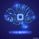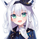7617 lượt xem

A regression line is simply a single line that best fits the data.

In the pinescript you can plot a linear regression line using the linreg function.

Here i share the entire calculation of the linear regression line, you are free to take the code and modify the functions in the script for creating your own kind of filter.

Hope you enjoy :)
Patreon: https://www.patreon.com/alexgrover

You can also check out some of the indicators I made for luxalgo : https://www.tradingview.com/u/LuxAlgo/#published-scripts

## Bình luậnthe linreg function on the trading view pine asks for an offset, which number in your formula is representing that?
Phản hồiCAB_Member
@CAB_Member, add the parameter offset as an input, then replace x by (x - offset) in line 16
Phản hồicmos
@cmos, Hi cmos, in python try using pandas with .rolling(window).corr where window is the correlation coefficient period, pandas allow you to compute any rolling statistic by using rolling(). The correlation coefficient from pine is calculated with : sma(y*x,length) - sma(y,length)*sma(x,length) divided by stdev(length*y,length)*stdev(length*x,length) where stdev is based on the naïve algorithm.
Phản hồiHi Alex this is very useful thanks!

I am trying to us the homodyne discriminator from ehlers ideal rsi, to set a variable length based on the price cycle for some of my indicators. this does work but the pinescript standard functions do not like dealing with a variable length, so i normally have to code out the functions... i am having such a problem with linreg, so finding your formula is very useful but it has the stock function 'sma' stdev' and 'correlation' which stops the code dead...

I wondered if you might be able to write out the stdev and the correlation functions in pinescript, if you have time.

Let me know what you think

Cheers

H
Phản hồi